Question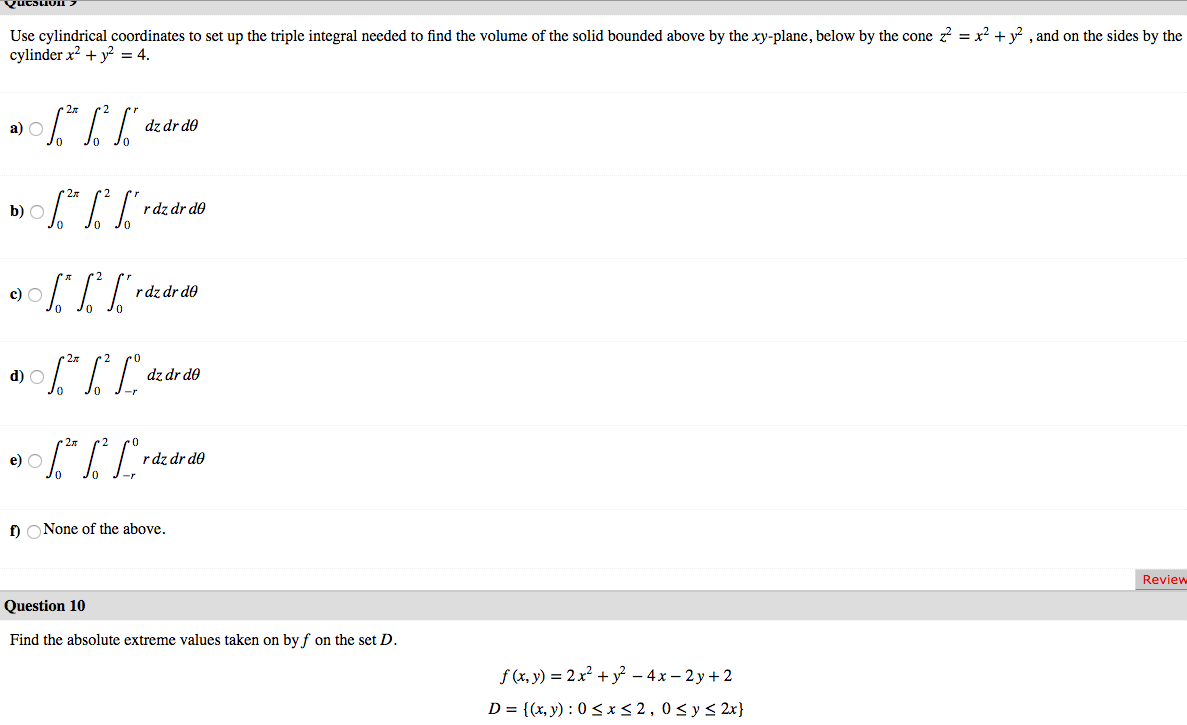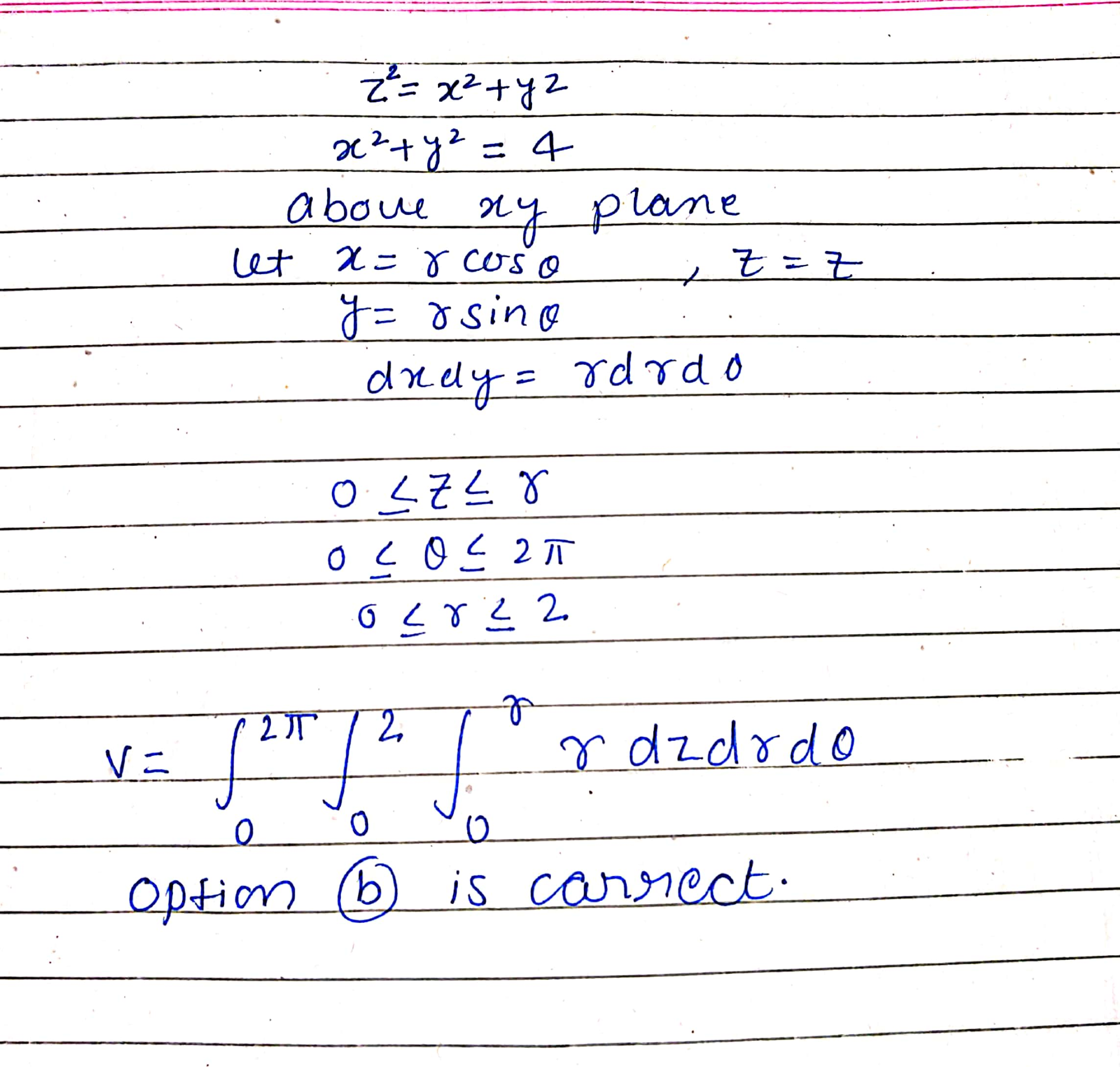according to the guidelines of HomeworkLib i have done first question please ask rest of the questions separately.....

#### Earn Coins

Coins can be redeemed for fabulous gifts.

Similar Homework Help Questions
• ### Set up, but do not evaluate, a triple integral in cylindrical coordinates that gives the volume...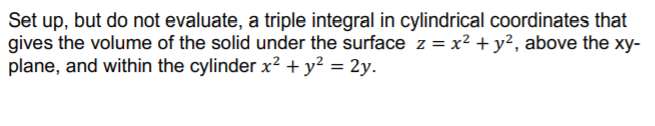Set up, but do not evaluate, a triple integral in cylindrical coordinates that gives the volume of the solid under the surface z = x2 + y2, above the xy- plane, and within the cylinder x2 + y2 = 2y.

• ### Use rectangular, cylindrical and spherical coordinates to set up the triple integrals representing the volume of the region bounded below by the xy plane, bounded above by the sphere with radius and...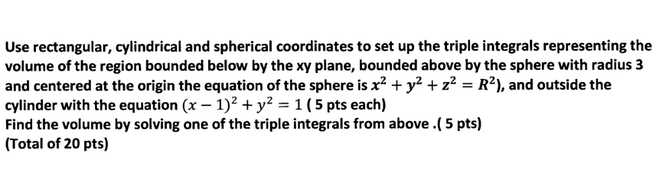Use rectangular, cylindrical and spherical coordinates to set up the triple integrals representing the volume of the region bounded below by the xy plane, bounded above by the sphere with radius and centered at the origin the equation of the sphere is x2 + y2 + z2-R2), and outside the cylinder with the equation (x - 1)2 +y2-1 (5 pts each) Find the volume by solving one of the triple integrals from above.( 5 pts) Total of 20 pts) Use...

• ### 16. Question Details LarCalc11 14.6.017. (3865000) Set up a triple integral for the volume of the...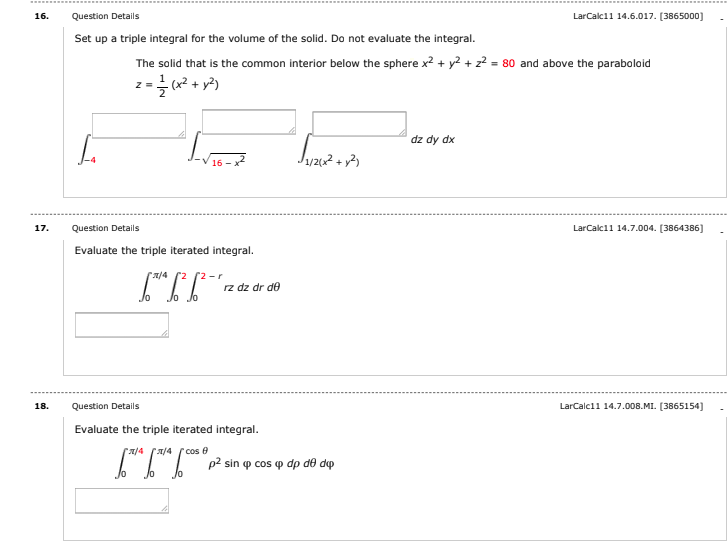16. Question Details LarCalc11 14.6.017. (3865000) Set up a triple integral for the volume of the solid. Do not evaluate the integral. The solid that is the common interior below the sphere x2 + y2 + 2+ = 80 and above the paraboloid z = {(x2 + y2) dz dy dx L J1/2012 + y2 Super 17. LarCalc11 14.7.004. (3864386] Question Details Evaluate the triple iterated integral. 6**6*6*2 2/4 2 2r rz dz dr de Jo lo 18. Question Details...

• ### 1. Use cylindrical coordinates to SET UP the integral for the volume of the portion of...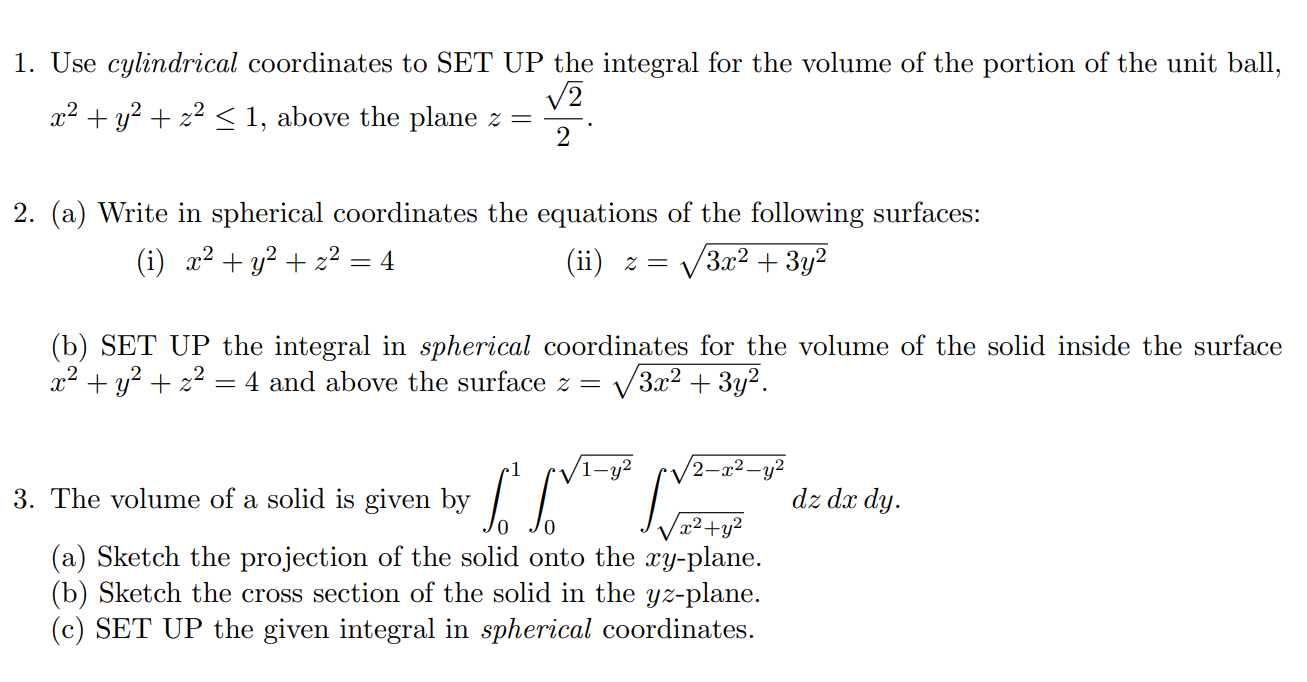1. Use cylindrical coordinates to SET UP the integral for the volume of the portion of the unit ball, 22 +232 + x2 < 1, above the plane z = 12 2. (a) Write in spherical coordinates the equations of the following surfaces: (i) x2 + y2 + x2 = 4 (ii) z = 3x2 + 3y2 (b) SET UP the integral in spherical coordinates for the volume of the solid inside the surface 22 + y2 + x2 =...

• ### Covert 1 = 83 84-** S*-**-»* dz dydx to an equivalent integral in cylindrical coordinates. Let...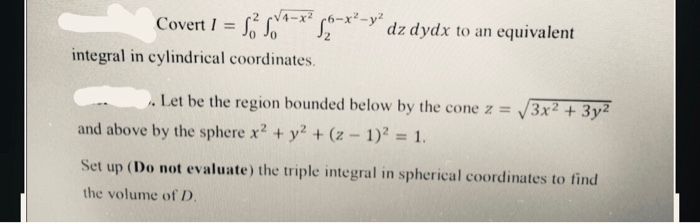Covert 1 = 83 84-** S*-**-»* dz dydx to an equivalent integral in cylindrical coordinates. Let be the region bounded below by the cone z = and above by the sphere x2 + y2 + (z - 1)2 = 1. |3x² + 3y² Set up (Do not evaluate) the triple integral in spherical coordinates to find the volume of D.

• ### Let D be the region bounded below by the cone z=x2+y2−−−−−−√ and above by the parabola z=2−x2−y2. Set up the triple integrals in cylindrical coordinates that give the volume of D using the following orders of integration: dzdrdθdzdrdθ.

Let D be the region bounded below by the cone z=x2+y2−−−−−−√ and above by the parabola z=2−x2−y2. Set up the triple integrals in cylindrical coordinates that give the volume of D using the following orders of integration: dzdrdθdzdrdθ.

• ### Use cylindrical coordinates to evaluate the triple integral ∭E √(x2+y2)dV where E is the solid bounded

Use cylindrical coordinates to evaluate the triple integral ∭E √(x2+y2)dV where E is the solid bounded by the circular paraboloid z = 1-1(x2+y2) and the xy -plane.

• ### 11. Evaluate S. 'S*(1 + 3x2 + 2y?) dx dy. 12. Find the volume in the...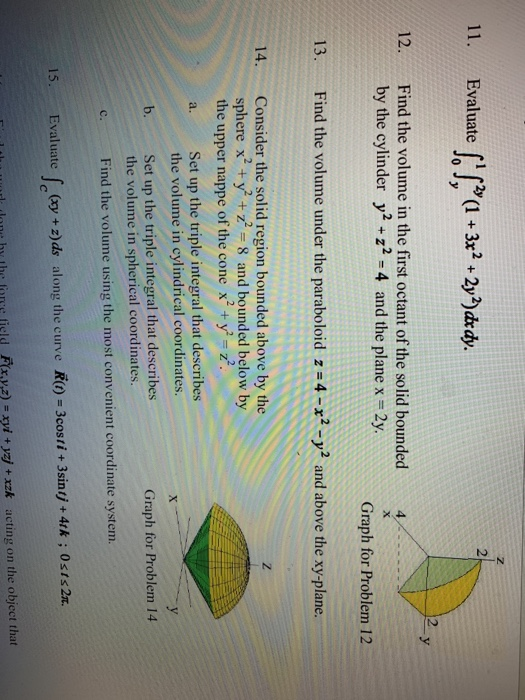11. Evaluate S. 'S*(1 + 3x2 + 2y?) dx dy. 12. Find the volume in the first octant of the solid bounded by the cylinder y2 + z2 = 4 and the plane x = 2y. Graph for Problem 12 13. Find the volume under the paraboloid z = 4 - x2 - y2 and above the xy-plane. N Consider the solid region bounded above by the sphere x + y + z = 8 and bounded below by the...

• ### Use cylindrical coordinates to evaluate the triple integral J Vi +y2 dV, where E is the...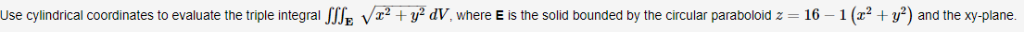Use cylindrical coordinates to evaluate the triple integral J Vi +y2 dV, where E is the solid bounded by the circular paraboloid z 16 -1(z2 +y2) and the xy-plane.

• ### A) solve this integral in cylindrical coordinates. b) set up the integral in spherical coordinates (without...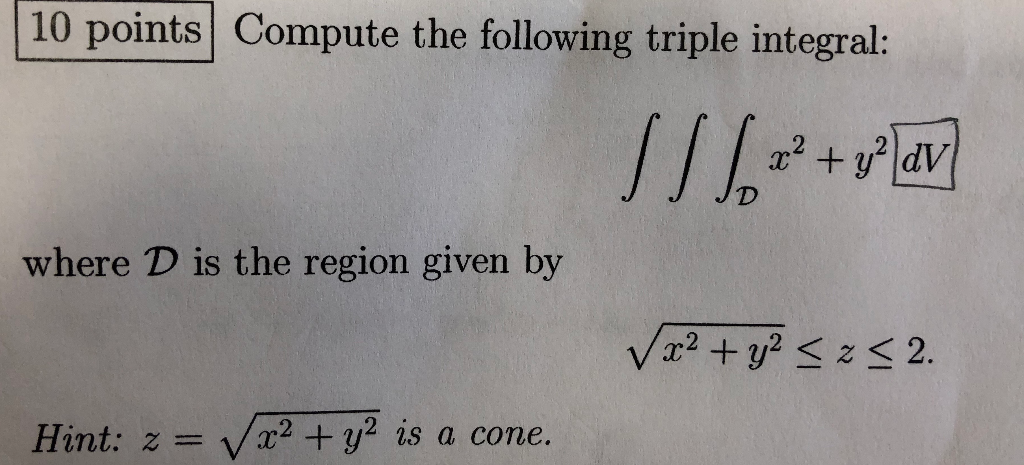A) solve this integral in cylindrical coordinates. b) set up the integral in spherical coordinates (without solving) 10 points Compute the following triple integral: 1/ 1.32 + plav JD where D is the region given by V x2 + y2 <2<2. Hint: z= V x2 + y2 is a cone.